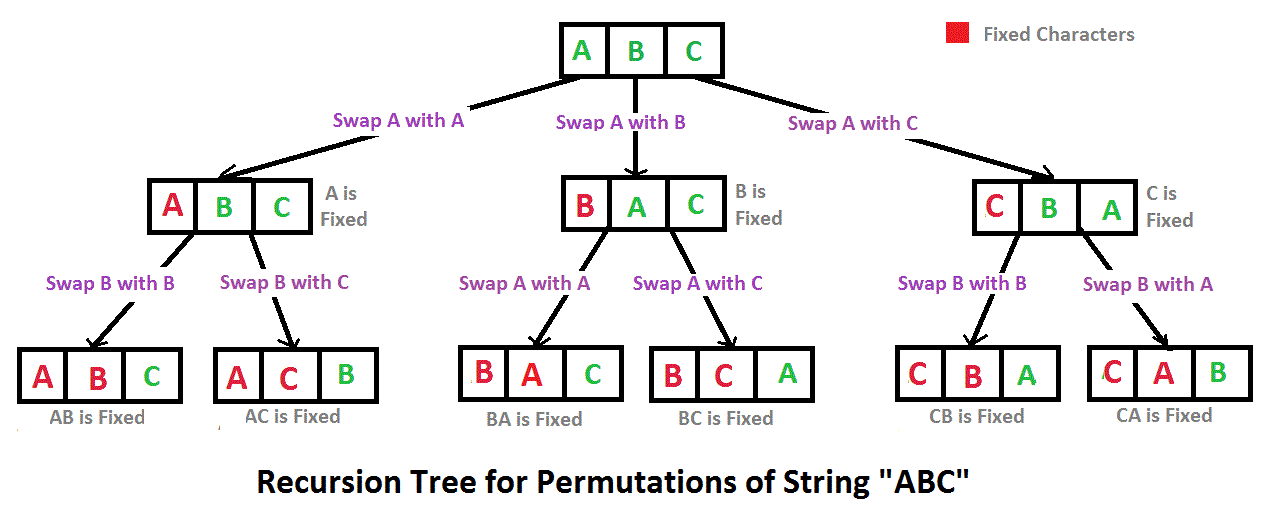# C# Program to print all permutations of a given string

• Difficulty Level : Hard
• Last Updated : 10 Dec, 2021

A permutation also called an “arrangement number” or “order,” is a rearrangement of the elements of an ordered list S into a one-to-one correspondence with S itself. A string of length n has n! permutation.

Source: Mathword(http://mathworld.wolfram.com/Permutation.html)

Below are the permutations of string ABC.
ABC ACB BAC BCA CBA CAB

Here is a solution that is used as a basis in backtracking.## C#

 `// C# program to print all permutations ``// of a given string. ``using` `System; `` ` `class` `GFG ``{ ``    ``/* Permutation function @param ``       ``str string to calculate permutation ``       ``for @param l starting index @param ``       ``r end index */``    ``private` `static` `void` `permute(String str, ``                                ``int` `l, ``int` `r) ``    ``{ ``        ``if` `(l == r) ``            ``Console.WriteLine(str); ``        ``else``        ``{ ``            ``for` `(``int` `i = l; i <= r; i++) ``            ``{ ``                ``str = swap(str, l, i); ``                ``permute(str, l + 1, r); ``                ``str = swap(str, l, i); ``            ``} ``        ``} ``    ``} `` ` `    ``/* Swap Characters at position ``       ``@param a string value @param ``       ``i position 1 @param j position 2 ``       ``@return swapped string */``    ``public` `static` `String swap(String a, ``                            ``int` `i, ``int` `j) ``    ``{ ``        ``char` `temp; ``        ``char``[] charArray = a.ToCharArray(); ``        ``temp = charArray[i] ; ``        ``charArray[i] = charArray[j]; ``        ``charArray[j] = temp; ``        ``string` `s = ``new` `string``(charArray); ``        ``return` `s; ``    ``} `` ` `// Driver Code ``public` `static` `void` `Main() ``{ ``    ``String str = ``"ABC"``; ``    ``int` `n = str.Length; ``    ``permute(str, 0, n-1); ``} ``} ``// This code is contributed by mits `

Output:

```ABC
ACB
BAC
BCA
CBA
CAB```

Time Complexity: O(n*n!) Note that there are n! permutations and it requires O(n) time to print a permutation.

Auxiliary Space: O(r – l)

Note: The above solution prints duplicate permutations if there are repeating characters in the input string. Please see the below link for a solution that prints only distinct permutations even if there are duplicates in input.
Print all distinct permutations of a given string with duplicates.
Permutations of a given string using STL

Another approach:

## C#

 `// C# program to implement``// the above approach``using` `System;``public` `class` `GFG{``  ` `static` `void` `permute(String s,``                    ``String answer)``{   ``    ``if` `(s.Length == 0)``    ``{``        ``Console.Write(answer + ``"  "``);``        ``return``;``    ``}``     ` `    ``for``(``int` `i = 0 ;i < s.Length; i++)``    ``{``        ``char` `ch = s[i];``        ``String left_substr = s.Substring(0, i);``        ``String right_substr = s.Substring(i + 1);``        ``String rest = left_substr + right_substr;``        ``permute(rest, answer + ch);``    ``}``}`` ` `// Driver code``public` `static` `void` `Main(String []args)``{    ``    ``String s;``    ``String answer=``""``;``     ` `    ``Console.Write(``    ``"Enter the string : "``);``    ``s = Console.ReadLine();``     ` `    ``Console.Write(``    ``"\nAll possible strings are : "``);``    ``permute(s, answer);``}``}``// This code is contributed by gauravrajput1 `

Output:

```Enter the string : abc
All possible strings are : abc  acb  bac  bca  cab  cba```

Time Complexity: O(n*n!) The time complexity is the same as the above approach, i.e. there are n! permutations and it requires O(n) time to print a permutation.

Auxiliary Space: O(|s|)

Please refer complete article on Write a program to print all permutations of a given string for more details!

My Personal Notes arrow_drop_up